Triangle Trigonometry: Help and Review Chapter Exam

Exam Instructions:

Choose your answers to the questions and click 'Next' to see the next set of questions. You can skip questions if you would like and come back to them later with the yellow "Go To First Skipped Question" button. When you have completed the practice exam, a green submit button will appear. Click it to see your results. Good luck!

Page 1

Question 1 1. Find the value of x, rounded to the nearest tenth.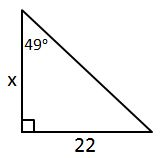Question 2 2. Find the distance between a and b.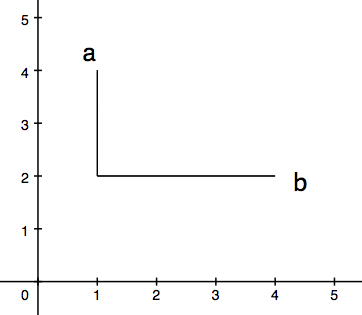Question 4 4. What is z in terms of x and y?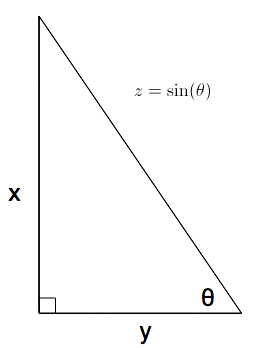Page 2

Question 9 9. What is x?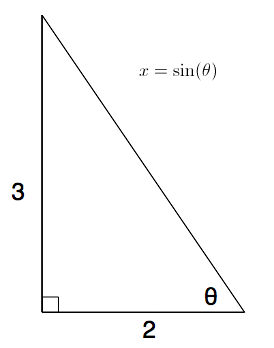Question 10 10. What is z in terms of x?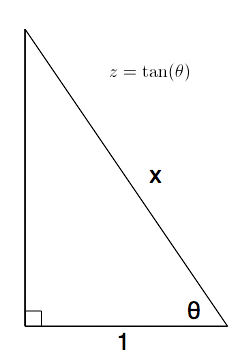Page 3

Question 11 11. Find the value of x, rounded to the nearest degree.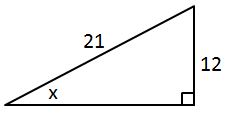Question 13 13. In the pictured triangle, what ratio represents the cosine of theta?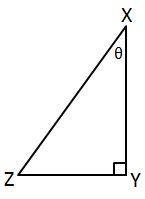Question 14 14. Jerry wants to buy a TV online, but the ad for the TV he wants does not say what the screen size is. He knows that TV screens are measured diagonally. The ad states the height and width of the screen, so he uses the Pythagorean Theorem to determine what the screen size is. What is the screen size of the TV that Jerry wants to buy?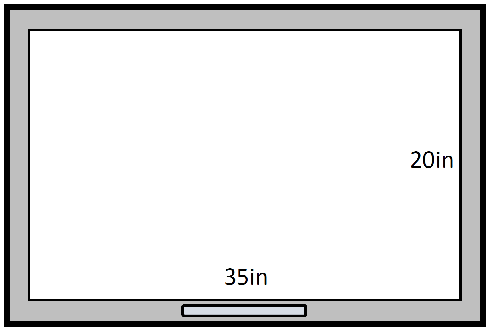Page 4

Question 17 17. Find the value of x, rounded to the nearest degree.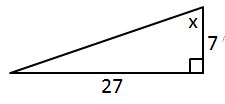Question 18 18. Find the value of x, rounded to the nearest tenth.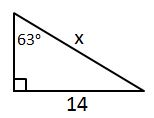Question 20 20. Find the value of x, rounded to the nearest tenth.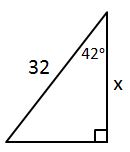Page 5

Question 21 21. What is x?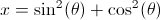Question 24 24. Which of the following is true?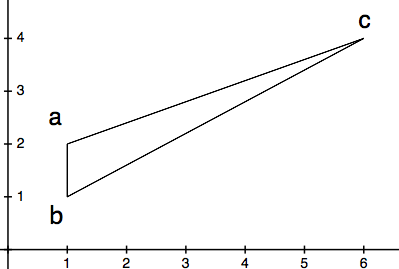Triangle Trigonometry: Help and Review Chapter Exam Instructions

Choose your answers to the questions and click 'Next' to see the next set of questions. You can skip questions if you would like and come back to them later with the yellow "Go To First Skipped Question" button. When you have completed the practice exam, a green submit button will appear. Click it to see your results. Good luck!

Support